Electron. J. Diff. Eqns., Vol. 2003(2003), No. 03, pp. 1-31.

### Singular solutions to Protter's problem for the 3-D wave equation involving lower order terms Myron. K. Grammatikopoulos, Tzvetan D. Hristov, & Nedyu I. Popivanov

Abstract:
In 1952, at a conference in New York, Protter formulated some boundary value problems for the wave equation, which are three-dimensional analogues of the Darboux problems (or Cauchy-Goursat problems) on the plane. Protter studied these problems in a 3-D domain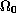, bounded by two characteristic cones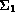and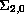, and by a plane region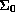. It is well known that, for an infinite number of smooth functions in the right-hand side, these problems do not have classical solutions. Popivanov and Schneider (1995) discovered the reason of this fact for the case of Dirichlet's and Neumann's conditions on: the strong power-type singularity appears in the generalized solution on the characteristic cone. In the present paper we consider the case of third boundary-value problem onand obtain the existence of many singular solutions for the wave equation involving lower order terms. Especifically, for Protter's problems in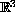it is shown here that for any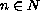there exists a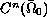-function, for which the corresponding unique generalized solution belongs to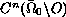and has a strong power type singularity at the point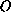. This singularity is isolated at the vertexof the characteristic cone. and does not propagate along the cone. For the wave equation without lower order terms, we presented the exact behavior of the singular solutions at the point.

Submitted May 27, 2002. Published January 2, 2003.
Math Subject Classifications: 35L05,35L20, 35D05, 35A20.
Key Words: Wave equation, boundary value problems, generalized solutions, singular solutions, propagation of singularities.

Show me the PDF file (364K), TEX file, and other files for this article.Myron K. Grammatikopoulos Department of Mathematics University of Ioannina 451 10 Ioannina, Greece email: mgrammat@cc.uoi.gr Tzvetan D. Hristov Institute of Mathematics and Informatics Bulgarian Academy of Sciences 1113 Sofia, Bulgaria email:tsvetan@fmi.uni-sofia.bg Nedyu I. Popivanov Department of Mathematics and Informatics University of Sofia 1164 Sofia, Bulgaria email: nedyu@fmi.uni-sofia.bg